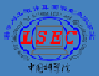```The laboratory is a multidisciplinary research center for scientific computation. The research of the laboratory focuses on the construction, analysis and implementation of efficient numerical methods and algorithms for solving practical and complex problems arising from various scientific and engineering applications. ``` ```The current research areas of the laboratory include: ``` `Finite element and boundary element methods;` `Nonlinear optimization and numerical algebra;` `Structure-preserving algorithms for dynamical systems;` `Computation of complex flows fluid flows and electromagnetics;` `Multi-physics, multi-scale computation of material properties; ` `Computational geometry and image processing;` `Computational biology and numerical relativity; ` `High performance scientific computing software and platforms.`